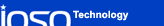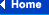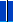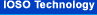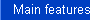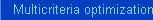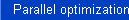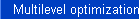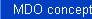### Multicriteria optimization in details

Multicriteria optimization can be done mathematically correctly only when some optimality principle is used. We use Pareto optimality principle, the essence of which is following. The multiobjective optimization problem solution is considered to be Pareto-optimal if there are no other solutions that are better in satisfying all of the objectives simultaneously. That is, there can be other solutions that are better in satisfying one or several objectives, but they must be worse than the Pareto-optimal solution in satisfying the remaining objectives.

In this case multiobjective optimization problem results in finding a full set of Pareto optimal solutions.

As a rule, it is impossible to find the full infinite set of Pareto optimal solutions for the particular real-life problems. For this reason the engineering multiobjective problem statement seeks to determine the finite subset of criteria-distinguishable Pareto optimal solutions.

It is vital to note, that at the initial stage of optimization process the accuracy of the response surfaces may be not so good due to the small number of points in the plan of experiment and the relatively large size of the current search area. However, during the optimization process, the number of points in the vicinity of the Pareto optimal points, is increased. At the same time, the size of the current search area is decreased. These trends are leading to a more accurate approximation of the objective functions and, hence, to the optimization process efficiency increase. Actually, during the optimization process, the information about the objective functions in the vicinity of the Pareto optimal points is permanently accumulated.

A number of heuristic procedures are developed for proposed optimization method efficiency increase as the information accumulates. These procedures are directed towards the adaptive change in the numbers of points in the plan of experiment and in the current search area. The adaptations affect the value of the normal distribution parameter and the rational selection in the basic optimization algorithm.

The main advantages of the proposed indirect multicriteria optimization method over traditional mathematical programming strategies lie in the possibility of the problem solving in case of nonconvex, discontinuous and stochastic goal functions and constraints; the unnecessity of considerable adaptation of the mathematical model for the optimization problem solving; the possibility to obtain a set of EP-optimal decisions under relatively small number of turns to the mathematical model; a significantly high probability of locating the global optimum in a multimodal design space. These advantages are the basis for the wide use of the proposed method in the real-life problems.

The main advantages of the proposed indirect multiobjective optimization method over traditional mathematical programming strategies is the following.

• The ability of solving the problems with nonconvex, discontinuous and stochastic objective functions and constraints;
• It is not necessary to considerable adapt the mathematical model for the optimization problem solving;
• The possibility to obtain a set of EP-optimal solutions using relatively small number of evaluations of the mathematical model;
• A significantly high probability of locating the global optimum in a multimodal design space.

These advantages are the basis for widely using of the proposed method in the real-life problems.